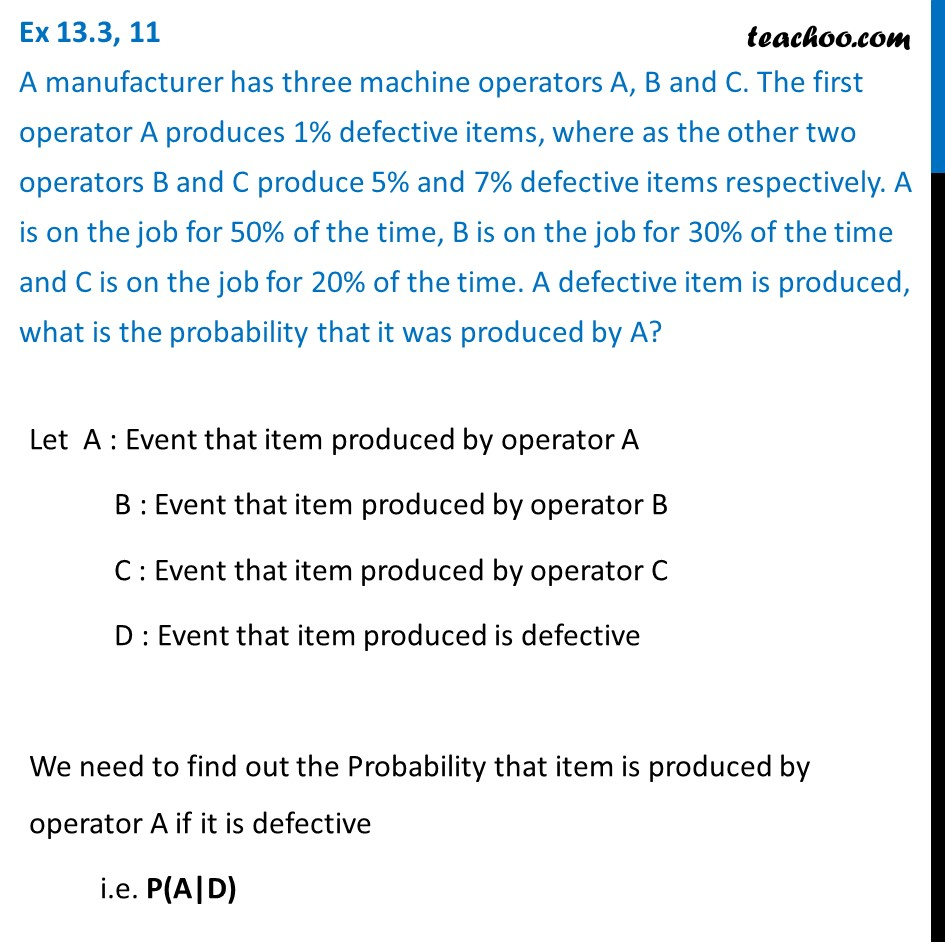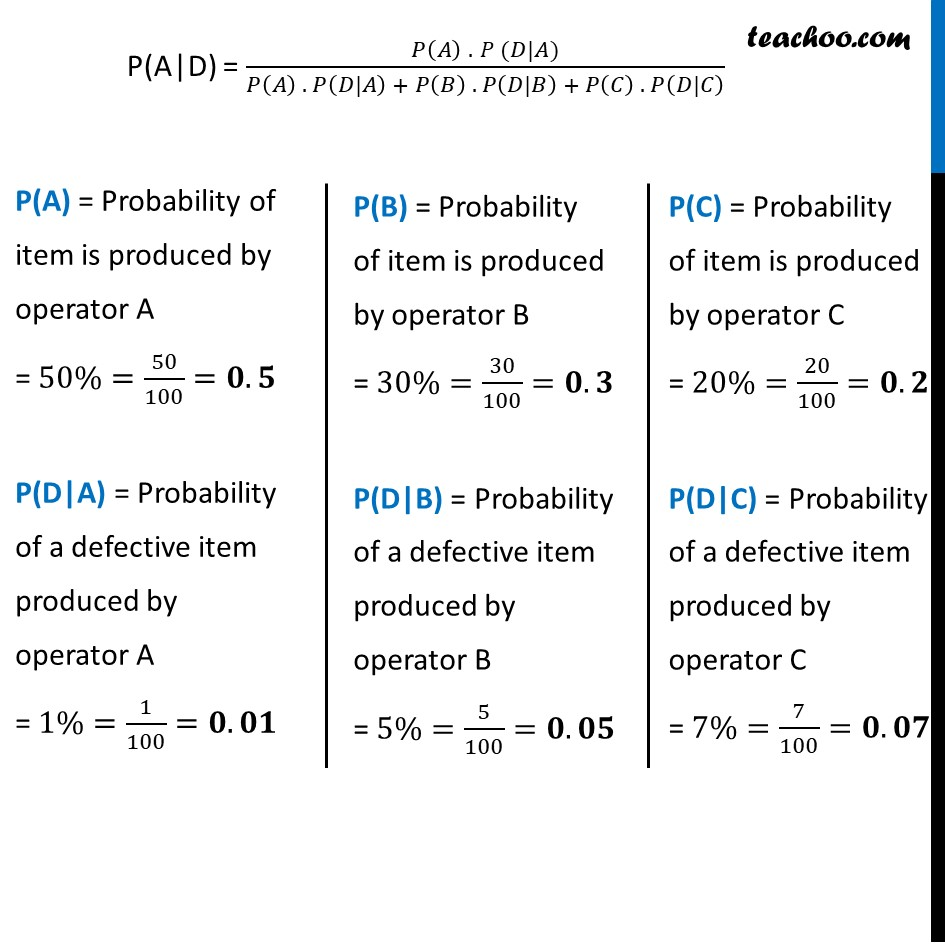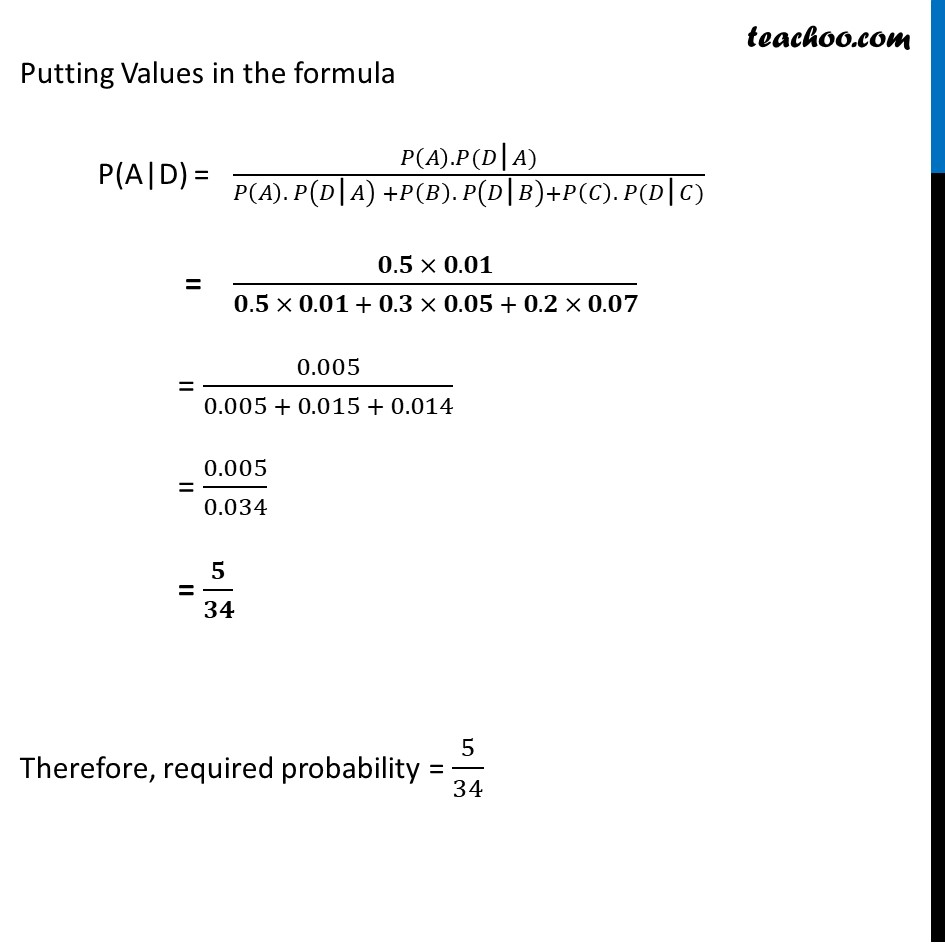Ex 13.3

Chapter 13 Class 12 Probability
Serial order wiseLearn in your speed, with individual attention - Teachoo Maths 1-on-1 Class

### Transcript

Ex 13.3, 11 A manufacturer has three machine operators A, B and C. The first operator A produces 1% defective items, where as the other two operators B and C produce 5% and 7% defective items respectively. A is on the job for 50% of the time, B is on the job for 30% of the time and C is on the job for 20% of the time. A defective item is produced, what is the probability that it was produced by A?Let A : Event that item produced by operator A B : Event that item produced by operator B C : Event that item produced by operator C D : Event that item produced is defective We need to find out the Probability that item is produced by operator A if it is defective i.e. P(A"|"D) P(A|D) = (𝑃(𝐴) ." " 𝑃(𝐷|𝐴))/(𝑃(𝐴) . 𝑃(𝐷|𝐴) + 𝑃(𝐵) . 𝑃(𝐷|𝐵) + 𝑃(𝐶) . 𝑃(𝐷|𝐶) ) "P(A)" = Probability of item is produced by operator A = 50%=50/100=𝟎.𝟓 P(D|A) = Probability of a defective item produced by operator A = 1%=1/100=𝟎.𝟎𝟏 "P(B)" = Probability of item is produced by operator B = 30%=30/100=𝟎.𝟑 P(D|B) = Probability of a defective item produced by operator B = 5%=5/100=𝟎.𝟎𝟓 "P(C)" = Probability of item is produced by operator C = 20%=20/100=𝟎.𝟐 P(D|C) = Probability of a defective item produced by operator C = 7%=7/100=𝟎.𝟎𝟕 Putting Values in the formula P(A|D) = (𝑃(𝐴). 𝑃(𝐷"|" 𝐴))/(𝑃(𝐴). 𝑃(𝐷"|" 𝐴) +𝑃(𝐵). 𝑃(𝐷"|" 𝐵)+𝑃(𝐶). 𝑃(𝐷"|" 𝐶)) = (𝟎.𝟓 × 𝟎.𝟎𝟏)/(𝟎.𝟓 × 𝟎.𝟎𝟏 + 𝟎.𝟑 × 𝟎.𝟎𝟓 + 𝟎.𝟐 × 𝟎.𝟎𝟕) = 0.005/(0.005 + 0.015 + 0.014) = 0.005/0.034 = 𝟓/𝟑𝟒 Therefore, required probability = 5/34